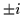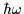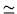Next: Dynamical moments and relative Up: Superdeformed bands in S Previous: Signature-splitting

## Signature-separation sensitive to the time-odd channels

A different situation takes place in configurations where both a neutron and a proton occupy unbalanced-signature states. In particular, four near-yrast 3131 configurations, shown in Figs. 6(a) and 7, group into two nearly degenerate pairs of bands having the same signature. Indeed, the r=+1 bands, 31-31- and 31+31+, are very close to one another, with the latter one lying slightly lower in energy, in accordance with the sign of the small signature splitting of the high-K 5/2(r=) orbitals. Note that the sudden deviation from regular behavior, seen in the latter band at=1.0-1.4MeV, is due to a poor HF convergence related to strong mixing of orbitals, see discussion in Sec. 3.4.

The second pair of degenerate 3131 bands corresponds to the signature r=-1, and is composed of the 31-31+ and 31+31- configurations, that are the mirror partners of one another in terms of the isospin. Therefore, irrespectively of the small signature splitting of the 5/2(r=) orbitals, these bands are almost perfectly degenerate. Again, due to the interactions between orbitals, at I10 and I14 one observes small irregularities reflecting poor HF convergence.

A remarkable feature obtained in the HF calculations is the fact that the pair of bands just mentioned, with r=-1, lies about 2MeV above the r=+1 pair. As opposed to the standard signature slitting effect, we my call these bands the signature-separated bands. Such a separation could not have been obtained in a phenomenological cranking model, i.e., the one using the Woods-Saxon or Nilsson potentials, because there the single-particle degeneracies immediately imply degeneracies of bands in many-body systems. Indeed, by putting one neutron and one proton into weakly split and non-interacting 5/2(r=) pair of orbitals, one should have obtained all the four 3131 bands strongly degenerate. Note that the separation of the r=-1 and r=+1 pairs of bands cannot be due to the deformation effect, because deformations of the four bands are very similar, see Fig. 8(a).

Strong separation of pairs of signature-degenerate bands results [40,41] from the self-consistent effects related to the time-odd components  in the HF mean fields. Odd particles induce the time-odd mean fields in odd and odd-odd nuclei, and similarly, odd particles in signature-unbalanced states induce the time-odd mean fields for certain configurations of even-even nuclei. In particular, when a neutron is put into the 5/2(r=+i) orbital, it creates, through the strong neutron-proton interaction which is inherent to any effective nuclear force, e.g., to the Skyrme force, a strong attractive component in the proton mean-field corresponding to the same, i.e. r=+i symmetry. Therefore, when the proton occupying the 5/2(r=+i) orbital is put into such a mean field, the total energy is significantly lowered. Of course, exactly the same mechanism applies for two particles occupying the 5/2(r=-i) orbitals. The proton mean field, generated by the 5/2(r=+i) neutron, does not influence the states of the r=-i symmetry, and therefore, adding then the 5/2(r=-i) proton does not influence the total energy. Hence, here the r=-1 bands are not affected by the time-odd interactions, (i.e., the interactions which give the time-odd mean fields through the HF averaging), while the r=+1 bands are significantly affected, and acquire an additional binding.

Obviously, the magnitude of the separation between the r=-1 and r=+1 bands crucially depends on the interaction strengths in time-odd channels. Unfortunately, the coupling constants corresponding to these channels  are not restricted by typical ground-state observables (masses, radii, etc.), that serve as experimental benchmarks with respect to which the force parameters are adjusted. Therefore, high spin effects, like the aforementioned r=-1 vs. r=+1 separation, that are manifestly sensitive to these unexplored channels of the interaction, could provide an extremely important information pertaining to basic properties of nuclear effective forces. Note that in 32S, the structure of the yrast line dramatically depends on the strength of the interaction in these channels, because the 3131 bands become yrast mostly due to the time-odd interaction.

By looking at similar quartets of bands, e.g., those corresponding to the 3333 and 3133 configurations, Figs. 6(a) and (c), respectively, one sees that the strength of interactions in the time-odd channels depends on the structure of the underlying orbitals. The signature splitting of the 1/2(r=) orbitals obscures the picture a little because it gives the splitting of the 33+33+ and 33-33- configurations, however, the centroid of these two configurations lies visibly below the perfectly degenerate pair of mirror partners 33-33+ and 33+33-. Hence, within the 1/2(r=) orbitals, the time-odd interaction is significantly weaker. Finally, there seems to be no such a non-diagonal interaction between the 1/2(r=) and 5/2(r=) orbitals, because the degeneracy pattern of the 3133 orbitals is completely different. Indeed, the standard, weakly split, two signature pairs appear, the lower one composed of the 31+33+ and 31-33+ configurations, and the higher one composed of the 31+33- and 31-33-configurations, in accordance with the sign of the signature splitting of the 1/2(r=) orbitals.

Results presented in this section indicate that the properly selected high-spin structures in a SD nucleus reflect the properties of the effective interaction in the time-odd channel. Quantitatively, in the restricted set of orbitals considered in 32S, the time-odd interaction amounts to an attractive force which acts between protons and neutrons occupying the same orbitals, i.e., orbitals having the same quantum numbers. Therefore, the discussed interaction channel has several features of the T=0 pairing interaction, although obviously the effects discussed here are not related to any collective pairing channels, but rather pertain to interactions in the particle-hole channel.Next: Dynamical moments and relative Up: Superdeformed bands in S Previous: Signature-splitting
Jacek Dobaczewski
1999-07-27